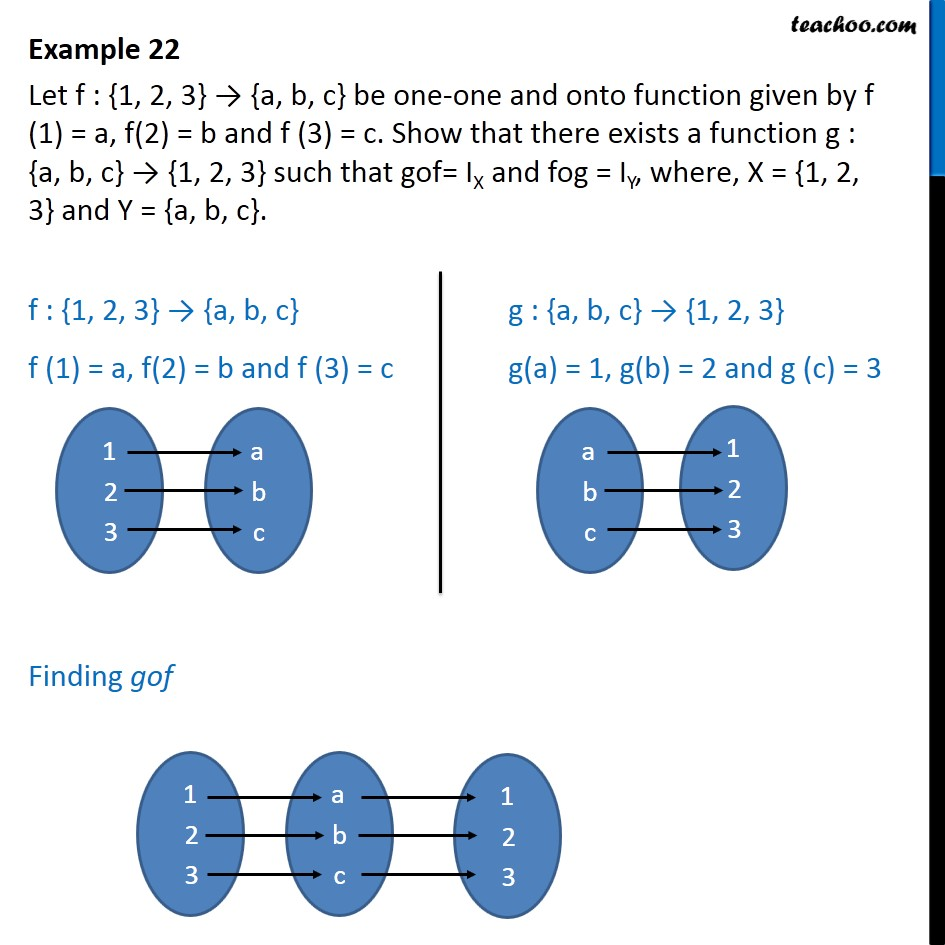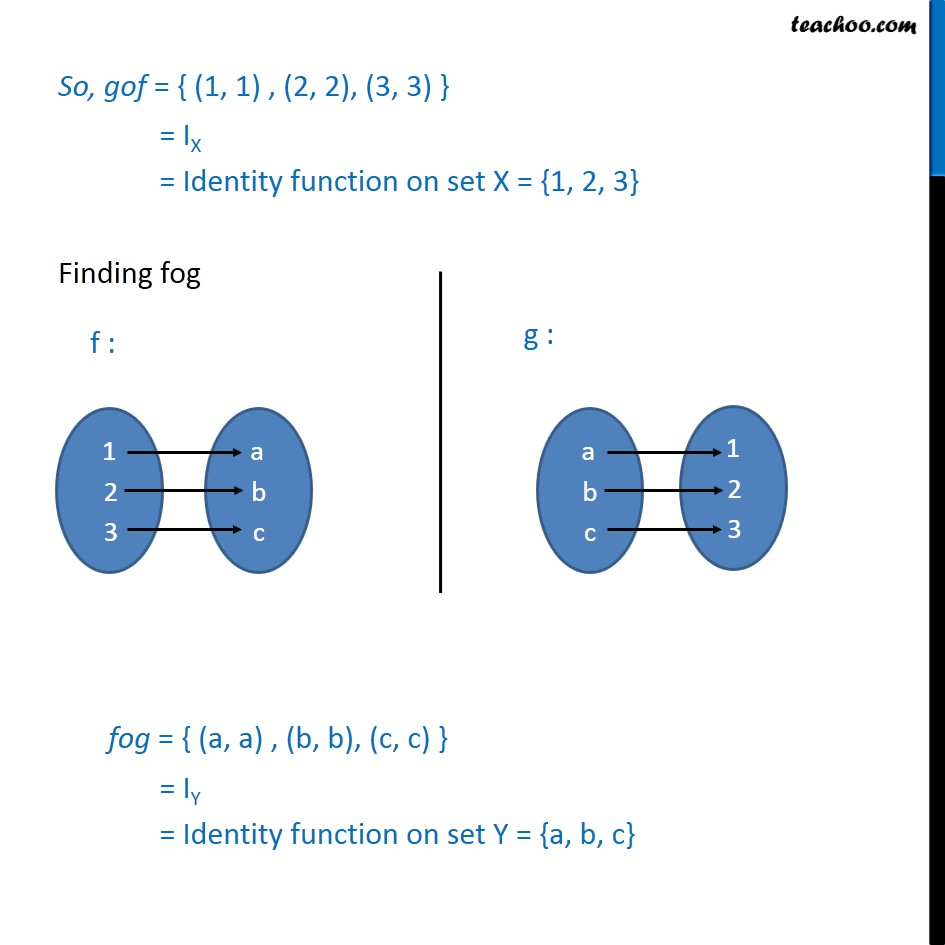Examples

Chapter 1 Class 12 Relation and Functions
Serial order wiseGet live Maths 1-on-1 Classs - Class 6 to 12

### Transcript

Example 22 Let f : {1, 2, 3} {a, b, c} be one-one and onto function given by f (1) = a, f(2) = b and f (3) = c. Show that there exists a function g : {a, b, c} {1, 2, 3} such that gof= IX and fog = IY, where, X = {1, 2, 3} and Y = {a, b, c}. Finding gof So, gof = { (1, 1) , (2, 2), (3, 3) } = IX = Identity function on set X = {1, 2, 3} Finding fog fog = { (a, a) , (b, b), (c, c) } = IY = Identity function on set Y = {a, b, c}